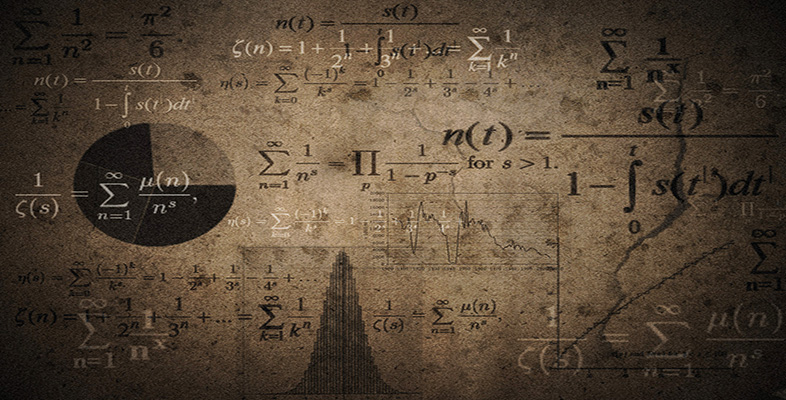Language, notation and formulas

Start this free course now. Just create an account and sign in. Enrol and complete the course for a free statement of participation or digital badge if available.

Free course

# 2.6.2 Try some yourself

## Activity 6

Read the following expression out aloud or write it out in full in words:

• (a) 3 × 4 + 3 × 5 = 3 (4 + 5).

• (a) Three times four, plus three times five equals three times the sum of four and five.

## Activity 7

Which mathematical operations are implied by these words when used in a mathematical sense?

• (a) Sum

• (b) Difference

• (c) Product

• (d) Quotient

State the operations in both words and symbols.

• (a) Sum implies addition or +.

• (b) Difference implies subtraction or −.

• (c) Product implies multiplication or ×.

• (d) Quotient implies division or ÷.

MU120_4M6